# OliNo

Renewable Energy

## 100 W incandescent lightbulb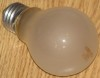This article gives interesting light parameters of an ordinary incandescent lamp of 100 W. It serves as a nice comparison article for the newer type of light bulbs like LED light bulbs. An Eulumdat file is added in this article.

See this overview for a comparison with other light bulbs.

### Summary measurement data

parameter meas. result remark
Color temperature 2758 K Warm white
Luminous intensity Iv 131 Cd
Beam angle 334 deg
Power P 101 W
Power Factor 1.0 For every 1 kWh net power consumed, there has been 0.0 kVAhr for reactive power.
Luminous flux 1503 lm
Luminous efficacy 15 lm/W The higher the power rating of incandescent light bulbs, the higher the temperature of the filament, and the higher the efficiency (to be compared with other incandescent light bulbs).
CRI_Ra 100 Color Rendering Index.
Coordinates chromaticity diagram x=0.4556 and y=0.4101
Fitting E27
D x H external dimensions 60 x 103 mm External dimensions of the light bulb.
D x H luminous area 60 x 76 mm Dimensions of the luminous area (used in Eulumdat file). This is equal to the size of the bulb itself (without the screw fitting).
General remarks The ambient temperature during the whole set of measurements was 24-27.5 deg C.

Warm up effect: no warm up time seen, the illumination value remains constant.

Voltage dependency: there is a linear dependency of illuminance and power consumption on the voltage.

The Eulumdat file can be found via this link.

### Eulumdat light diagram

An interesting graph is the light diagram, indicating the intensity in the C0-C180 and the C90-C270 plane. This light diagram below comes from the program Qlumedit, that extracts these diagrams from an Eulumdat file.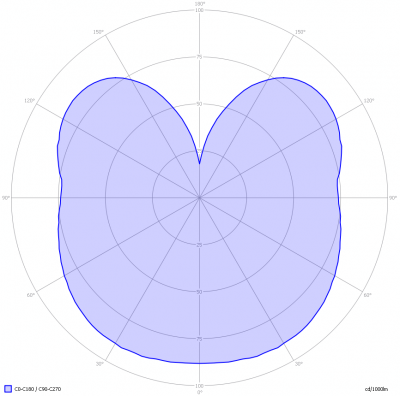The light diagram giving the radiation pattern.

It indicates the luminous intensity around the light bulb. This light diagram is symmetrical around the z-axis (hence the C0-C180 plane gives the same result as the C90-C270 plane).

The unit is Cd/1000lm, meaning the intensity in Cd assuming there would be 1000 lumen in the measured light bulb. This enables comparing different types of light bulbs.

### Illuminance Ev at 1 m distance, or luminous intensity Iv

Herewith the plot of the averaged luminous intensity Iv as a function of the inclination angle with the light bulb.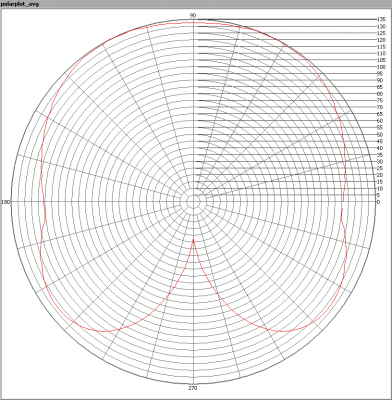The radiation pattern of the light bulb.

This radiation pattern is the same as the one given earlier. This is because in this light bulb’s case, the radiation pattern is symmetric around the z-axis, meaning that the averaged pattern given here is the same as the extraction of the Eulumdat file.

These averaged values are used (later) to compute the lumen output.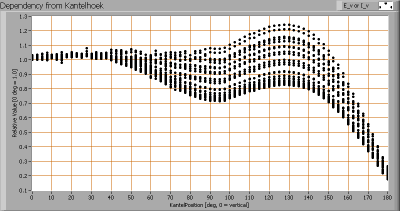Intensity data of every measured turn angle at each inclination angle.

This plot shows per inclination angle the intensity measurement results for each turn angle at that inclination angle. So at 90 degrees inclination angle, all the measurement results of illuminance for all measured turn angles are in the range of 70-110 % of the illuminance value at 0 degrees inclination angle.

When using the average values per inclination angle, the beam angle can be computed, being 334 degrees.

### Luminous flux

With the averaged illuminance data at 1 m distance, taken from the graph showing the averaged radiation pattern, it is possible to compute the luminous flux.

The result of this computation for this light spot is a luminous flux of 1503 lm.

### Luminous efficacy

The luminous flux being 1503 lm, and the power of the lightbulb being 101 W, yields a luminous efficacy of 15 lm/W.

A power factor of 1.0 means that for every 1 kWh net power consumed, a reactive component of 0.0 kVAr was needed.

 Light bulb voltage 230 V Light bulb current 441 mA Power P 101 W Apparent power S 101 VA Power factor 1.0

Of this light bulb the voltage across ad the resulting current through it are measured and graphed.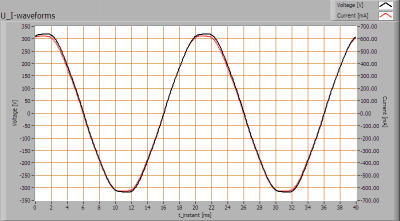Voltage across and current through the lightbulb
The current has the exact same phase and form as the voltage. This is why the power factor equals 1.
Also the power spectrum of the current is determined.Powerspectrum of the current
This powerspectrum only shows the ground harmonic and (virtually) no higher harmonic currents.

### Color temperature and Spectral power distribution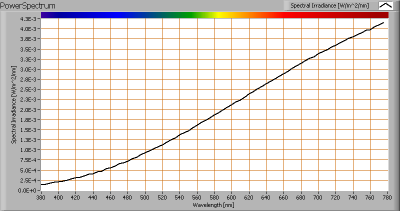The spectral power distribution of this light bulb.

The measured color temperature is about 2758 K which is deep warm white.

This color temperature is measured straight underneath the light bulb. Below a graph showing the color temperature for different inclination angles.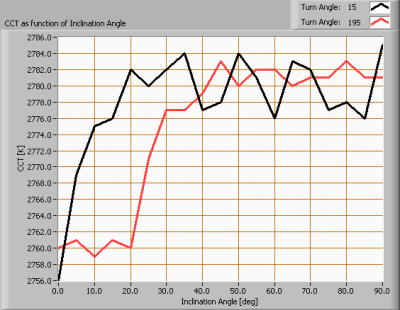Color temperature as a function of inclination angle.

The colortemperature is given for inclination angles up to 90 degrees, no measurements were taken beyond that.

The value remains constant within 1 %.

### Chromaticity diagram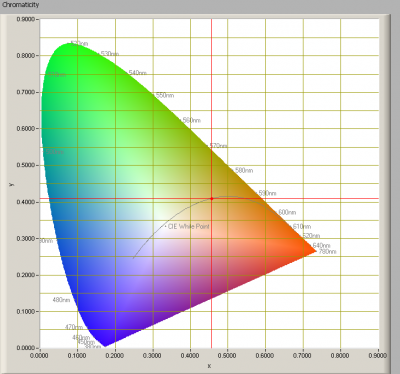The chromaticity space and the position of the lamp’s color coordinates in it.

The light coming from this lamp is on top of the Planckian Locus (the black path in the graph).

Its coordinates are x=0.4556 and y=0.4101.

### Color Rendering Index (CRI) or also Ra

Herewith the image showing the CRI as well as how well different colors are represented (rendered). The higher the number, the better the resemblance with the color when a black body radiator would have been used (the sun, or an incandescent lamp).

Each color has an index Rx, and the first 8 indexes (R1 .. R8) are averaged to compute the Ra which is equivalent to the CRI.CRI of the light2 of this lightbulb.

The value of 100 is higher than 80 which is considered a minimum value for indoor usage.

Note: the chromaticity difference is 0.0002 indicates the distance to the Planckian Locus. Its value is lower than 0.0054, which means that the calculated CRI result is meaningful.

### Voltage dependency

The dependency of a number of lamp parameters on the lamp voltage is determined. For this, the lamp voltage has been varied and its effect on the following lamp parameters measured: illuminance E_v [lx], color temperature CT or correlated color temperature CCT [K], the lamppower P [W] and the luminous efficacy [lm/W].Lamp voltage dependencies of certain light bulb parameters, where the value at 230 V is taken as 100 %.

The consumed power and the illuminance vary largely when the voltage varies between 200 – 250 V. This variation is linear.

When the voltage at 230 V varies with + and – 5 V, then the illuminance varies with less than + and – 8 %, which might be visible when the voltage changes abruptly.

### Warm up effects

After switch on of a cold lamp, the effect of heating up of the lamp is measured on illuminance E_v [lx], color temperature CT or correlated color temperature CCT [K], the lamppower P [W] and the luminous efficacy [lm/W].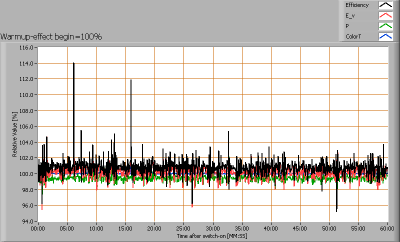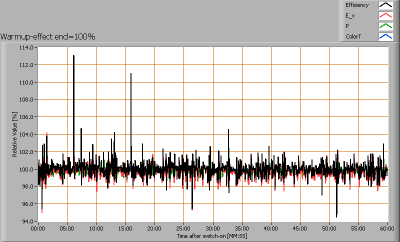Effect of warming up on different light bulb parameters. At top the 100 % level is put at begin, and at bottom at the end.

No variation in the illuminance nor power consumed, there is no warmup time.